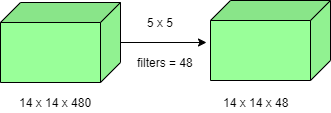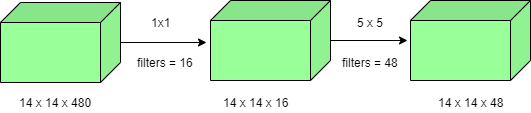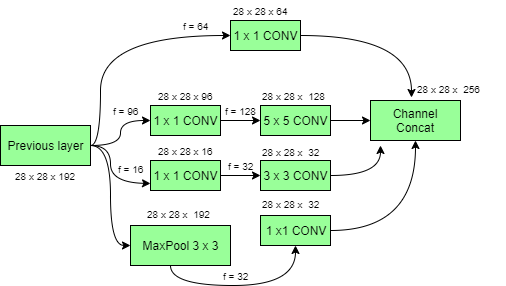Skip to content
Related Articles
ML | Inception Network V1
• Last Updated : 23 Jan, 2020

Inception net achieved a milestone in CNN classifiers when previous models were just going deeper to improve the performance and accuracy but compromising the computational cost. The Inception network, on the other hand, is heavily engineered. It uses a lot of tricks to push performance, both in terms of speed and accuracy. It is the winner of the ImageNet Large Scale Visual Recognition Competition in 2014, an image classification competition, which has a significant improvement over ZFNet (The winner in 2013), AlexNet (The winner in 2012) and has relatively lower error rate compared with the VGGNet (1st runner-up in 2014).

The major issues faced by deeper CNN models such as VGGNet were:

• Although, previous networks such as VGG achieved a remarkable accuracy on the ImageNet dataset, deploying these kinds of models is highly computationally expensive because of the deep architecture.
• Very deep networks are susceptible to overfitting. It is also hard to pass gradient updates through the entire network.

Before digging into Inception Net model, it’s essential to know an important concept that is used in Inception network:

1 X 1 convolution: A 1×1 convolution simply maps an input pixel with all its respective channels to an output pixel. 1×1 convolution is used as a dimensionality reduction module to reduce computation to an extent.

• For instance, we need to perform 5×5 convolution without using 1×1 convolution as below:Number of operations involved here is (14×14×48) × (5×5×480) = 112.9M

• Using 1×1 convolution:Number of operations for 1×1 convolution = (14×14×16) × (1×1×480) = 1.5M
Number of operations for 5×5 convolution = (14×14×48) × (5×5×16) = 3.8M
After addition we get, 1.5M + 3.8M = 5.3M

Which is immensely smaller than 112.9M ! Thus, 1×1 convolution can help to reduce model size which can also somehow help to reduce the overfitting problem.

Inception model with dimension reductions:Deep Convolutional Networks are computationally expensive. However, computational costs can be reduced drastically by introducing a 1 x 1 convolution. Here, the number of input channels is limited by adding an extra 1×1 convolution before the 3×3 and 5×5 convolutions. Though adding an extra operation may seem counter-intuitive but 1×1 convolutions are far cheaper than 5×5 convolutions. Do note that the 1×1 convolution is introduced after the max-pooling layer, rather than before. At last, all the channels in the network are concatenated together i.e. (28 x 28 x (64 + 128 + 32 + 32)) = 28 x 28 x 256.

GoogLeNet Architecture of Inception Network:
This architecture has 22 layers in total! Using the dimension-reduced inception module, a neural network architecture is constructed. This is popularly known as GoogLeNet (Inception v1). GoogLeNet has 9 such inception modules fitted linearly. It is 22 layers deep (27, including the pooling layers). At the end of the architecture, fully connected layers were replaced by a global average pooling which calculates the average of every feature map. This indeed dramatically declines the total number of parameters.

Thus, Inception Net is a victory over the previous versions of CNN models. It achieves an accuracy of top-5 on ImageNet, it reduces the computational cost to a great extent without compromising the speed and accuracy.My Personal Notes arrow_drop_up